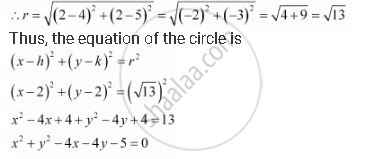# Find the Equation of a Circle with Centre (2, 2) and Passes Through the Point (4, 5). - Mathematics

Find the equation of a circle with centre (2, 2) and passes through the point (4, 5).

#### Solution

The centre of the circle is given as (hk) = (2, 2).

Since the circle passes through point (4, 5), the radius (r) of the circle is the distance between the points (2, 2) and (4, 5).Concept: Concept of Circle
Is there an error in this question or solution?
Chapter 11: Conic Sections - Exercise 11.1 [Page 241]

#### APPEARS IN

NCERT Class 11 Mathematics
Chapter 11 Conic Sections
Exercise 11.1 | Q 14 | Page 241

Share